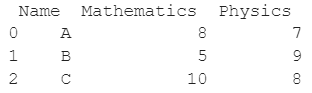# How To Get Column Index From Column Name In Python Pandas?

A DataFrame is the primary data structure of the Pandas library and is commonly used for storing and working with tabular data in Python. A common operation that could be performed on such data is to get a list of a column or row in order to extract information from it.

To start working with Pandas, we first need to import it by using the below statement:

``import pandas as pd``

## Running Example

Let us understand this operation with the help of an example. Consider the following DataFrame containing 3 students with names A, B, and C and their corresponding marks (out of 10) for two subjects, Mathematics and Physics.Code snippet for generating the above DataFrame:

``````import pandas as pd
# Dictionary for our data
data = {'Name' : ['A', 'B', 'C'], 'Mathematics' : [8, 5, 10], 'Physics' : [7, 9, 8]}
# DataFrame for the dictionary
df = pd.DataFrame(data)
# Printing the DataFrame
print(df)``````

Here, data is a dictionary, we created to initialize the DataFrame. For this, we use the DataFrame() function of the Pandas library which takes the dictionary as an argument and returns the required DataFrame.

Now let us say that for some reason, we want to get the index of the column Physics of this DataFrame df, the resulting output will look as follows:

2

Let us look at different ways of performing this operation on a given DataFrame :

## Get index of dataframe column using the columns.get_loc() function in Pandas

In this method, we use the DataFrame.columns.get_loc() function to get the index of a particular column of an existing Pandas DataFrame based on the name (or label) of that column.

The column of interest is Physics here. The df.columns returns the columns of the DataFrame and when the .get_loc() function is applied on this resulting object along with the parameter passed as the label of desired column, Physics here, we get the index of this column. Let us take a look at the code and corresponding output for this method.

``````import pandas as pd
# Dictionary for our data
data = {'Name' : ['A', 'B', 'C'], 'Mathematics' : [8, 5, 10], 'Physics' : [7, 9, 8]}
# DataFrame for the dictionary
df = pd.DataFrame(data)
# Getting the index of column named as Physics
idx = df.columns.get_loc('Physics')
# Printing the index
print(idx)``````

Output :

2

If we try to get the index of a column that does not exist in the DataFrame, we get an error. Let us look at the code and corresponding output for this operation.

``````import pandas as pd
# Dictionary for our data
data = {'Name' : ['A', 'B', 'C'], 'Mathematics' : [8, 5, 10], 'Physics' : [7, 9, 8]}
# DataFrame for the dictionary
df = pd.DataFrame(data)
# Getting the index of column named as History
idx = df.columns.get_loc(â€˜Historyâ€™)
# Printing the index
print(idx)``````

Output :

ValueError: 'History' is not in list

## Get index of dataframe column using the list().index() function in Pandas

In this method, we use the list(),index() function to get the index of a particular column of an existing Pandas DataFrame based on the name (or label) of that column.

The column of interest is Physics here. The df.columns returns the columns of the DataFrame. When df.columns is passed as a parameter to the list() function, it returns a type casted list.

After applying the index() function with the parameter passed as the label of the desired column, Physics here, we get the index of this column.

Let us take a look at the code and corresponding output for this method.

``````import pandas as pd
# Dictionary for our data
data = {'Name' : ['A', 'B', 'C'], 'Mathematics' : [8, 5, 10], 'Physics' : [7, 9, 8]}
# DataFrame for the dictionary
df = pd.DataFrame(data)
# Getting the index of column named as Physics
idx = list(df.columns).index('Physics')
# Printing the index
print(idx)``````

Output :

2

If we try to get the index of a column that does not exist in the DataFrame, we get an error. Let us look at the code and corresponding output for this operation.

``````import pandas as pd
# Dictionary for our data
data = {'Name' : ['A', 'B', 'C'], 'Mathematics' : [8, 5, 10], 'Physics' : [7, 9, 8]}
# DataFrame for the dictionary
df = pd.DataFrame(data)
# Getting the index of column named as History
idx = list(df.columns).index('History')
# Printing the index
print(idx)``````

Output :

ValueError: 'History' is not in list

## Conclusion

In this topic, we have learned to get the index of a column based on the column name (or label) of an existing Pandas DataFrame, following a running example of test scores of students in different subjects, thus giving us an intuition of how this concept could be applied in real-world situations.

Trending